# AP Calculus AB : Understanding continuity in terms of limits

## Example Questions

### Example Question #1 : Understanding Continuity In Terms Of Limits

If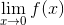exists,

We cannot conclude any of the other answers.must be continuous at allvalues.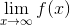exists.must be continuous at.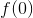exists andWe cannot conclude any of the other answers.

Explanation:

Unless we are explicitly told so, via graph, information, or otherwise, we cannot assumeis continuous atunless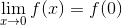, which is required forto be continuous at.

We cannot assume anything about the existence of, because we do not know whatis, or its end behavior.

### Example Question #2 : Understanding Continuity In Terms Of Limits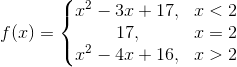Which of the following is equal to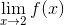?does not exist.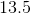does not exist.

Explanation:

The limit of a function asapproaches a value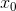exists if and only if the limit from the left is equal to the limit from the right; the actual value of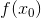is irrelevant. Since the function is piecewise-defined, we can determine whether these limits are equal by finding the limits of the individual expressions. These are both polynomials, so the limits can be calculated using straightforward substitution: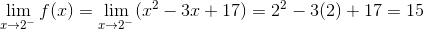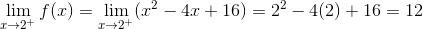does not exist, because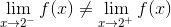.

### Example Question #3 : Understanding Continuity In Terms Of Limits

Determine any points of discontinuity for the function: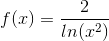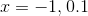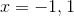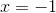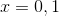Explanation:

For a function to be continuous the following criteria must be met:

1.  The function must exist at the point (no division by zero, asymptotic behavior, negative logs, or negative radicals).
2. The limit must exist.
3. The point must equal the limit. (Symbolically,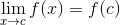).

It is easiest to first find any points where the function is undefined. Since our function involves a fraction and a natural log, we must find all points in the domain such that the natural log is less than or equal to zero, or points where the denominator is equal to zero.

To find the values that cause the natural log to be negative we set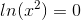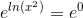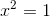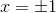Therefore, those x values will yield our points of discontinuity. Normally, we would find values where the natural log is negative; however, for allthe function is positive.